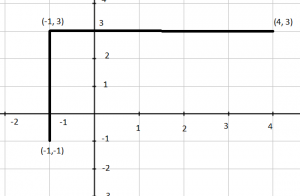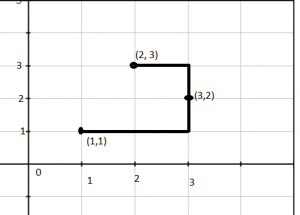# Number of horizontal or vertical line segments to connect 3 points

• Difficulty Level : Medium
• Last Updated : 15 Jun, 2022

Given three points on the x-y coordinate plane. You need to find the no. of line segments formed by making a polyline passing through these points. (Line segment can be vertically or horizontally aligned to the coordinate axis)
Examples :

```Input : A  = {-1, -1}, B = {-1, 3}, C = {4, 3}
Output :   2
Expantaion:``````There are two segments in this polyline.
Input :A = {1, 1}, B = {2, 3} C = {3, 2}
Output : 3```` `

The result is one if all points are on x axis or y axis. The result is 2 if points can form L shape. L shape is formed if any of the three points can be used as a joining point. Otherwise answer is 3.

## C++

 `// CPP program to find number of horizontal (or vertical``// line segments needed to connect three points.``#include ``using` `namespace` `std;` `// Function to check if the third point forms a``// rectangle with other two points at corners``bool` `isBetween(``int` `a, ``int` `b, ``int` `c)``{``    ``return` `min(a, b) <= c && c <= max(a, b);``}` `// Returns true if point k can be used as a joining``// point to connect using two line segments``bool` `canJoin(``int` `x[], ``int` `y[], ``int` `i, ``int` `j, ``int` `k)``{``    ``// Check for the valid polyline with two segments``    ``return` `(x[k] == x[i] || x[k] == x[j]) &&``                ``isBetween(y[i], y[j], y[k]) ||``        ``(y[k] == y[i] || y[k] == y[j]) &&``                ``isBetween(x[i], x[j], x[k]);``}` `int` `countLineSegments(``int` `x[], ``int` `y[])``{``    ``// Check whether the X-coordinates or``    ``// Y-cocordinates are same.``    ``if` `((x == x && x == x) ||``        ``(y == y && y == y))``        ``return` `1;` `    ``// Iterate over all pairs to check for two``    ``// line segments``    ``else` `if` `(canJoin(x, y, 0, 1, 2) ||``            ``canJoin(x, y, 0, 2, 1) ||``            ``canJoin(x, y, 1, 2, 0))``        ``return` `2;` `    ``// Otherwise answer is three.``    ``else``        ``return` `3;``}` `// Driver code``int` `main()``{``    ``int` `x, y;``    ``x = -1; y = -1;``    ``x = -1; y = 3;``    ``x = 4; y = 3;``    ``cout << countLineSegments(x, y);``    ``return` `0;``}`

## Java

 `// Java program to find number of horizontal``// (or vertical line segments needed to``// connect three points.``import` `java.io.*;` `class` `GFG {``    ` `// Function to check if the third``// point forms a rectangle with``// other two points at corners``static` `boolean` `isBetween(``int` `a, ``int` `b, ``int` `c)``{``    ``return` `(Math.min(a, b) <= c &&``                    ``c <= Math.max(a, b));``}` `// Returns true if point k can be``// used as a joining point to connect``// using two line segments``static` `boolean` `canJoin(``int` `x[], ``int` `y[],``                        ``int` `i, ``int` `j, ``int` `k)``{``    ``// Check for the valid polyline``    ``// with two segments``    ``return` `(x[k] == x[i] || x[k] == x[j]) &&``                ``isBetween(y[i], y[j], y[k]) ||``                ``(y[k] == y[i] || y[k] == y[j]) &&``                ``isBetween(x[i], x[j], x[k]);``}` `static` `int` `countLineSegments(``int` `x[], ``int` `y[])``{``    ``// Check whether the X-coordinates or``    ``// Y-cocordinates are same.``    ``if` `((x[``0``] == x[``1``] && x[``1``] == x[``2``]) ||``        ``(y[``0``] == y[``1``] && y[``1``] == y[``2``]))``        ``return` `1``;` `    ``// Iterate over all pairs to check for two``    ``// line segments``    ``else` `if` `(canJoin(x, y, ``0``, ``1``, ``2``) ||``            ``canJoin(x, y, ``0``, ``2``, ``1``) ||``            ``canJoin(x, y, ``1``, ``2``, ``0``))``        ``return` `2``;` `    ``// Otherwise answer is three.``    ``else``        ``return` `3``;``}` `// Driver code``public` `static` `void` `main (String[] args) {` `    ``int` `x[]=``new` `int``[``3``], y[]=``new` `int``[``3``];``    ` `    ``x[``0``] = -``1``; y[``0``] = -``1``;``    ``x[``1``] = -``1``; y[``1``] = ``3``;``    ``x[``2``] = ``4``; y[``2``] = ``3``;``    ` `    ``System.out.println(countLineSegments(x, y));``    ``}``    ` `    ` `}` `// This code is contributed by vt_m`

## Python3

 `# Python program to find number``# of horizontal (or vertical``# line segments needed to``# connect three points.` `import` `math` `# Function to check if the``# third point forms a``# rectangle with other``# two points at corners``def` `isBetween(a, b, c) :` `    ``return` `min``(a, b) <``=` `c ``and` `c <``=` `max``(a, b)` ` ` `# Returns true if point k``# can be used as a joining``# point to connect using``# two line segments``def` `canJoin( x, y, i, j, k) :` `    ``# Check for the valid polyline``    ``# with two segments``    ``return` `(x[k] ``=``=` `x[i] ``or` `x[k] ``=``=` `x[j]) ``and` `isBetween(y[i], y[j], y[k]) ``or` `(y[k] ``=``=` `y[i] ``or` `y[k] ``=``=` `y[j]) ``and` `isBetween(x[i], x[j], x[k])` ` ` `def` `countLineSegments( x, y):` `    ``# Check whether the X-coordinates or``    ``# Y-cocordinates are same.``    ``if` `((x[``0``] ``=``=` `x[``1``] ``and` `x[``1``] ``=``=` `x[``2``]) ``or``        ``(y[``0``] ``=``=` `y[``1``] ``and` `y[``1``] ``=``=` `y[``2``])):``        ``return` `1`` ` `    ``# Iterate over all pairs to check for two``    ``# line segments``    ``elif` `(canJoin(x, y, ``0``, ``1``, ``2``) ``or``            ``canJoin(x, y, ``0``, ``2``, ``1``) ``or``            ``canJoin(x, y, ``1``, ``2``, ``0``)):``        ``return` `2`` ` `    ``# Otherwise answer is three.``    ``else``:``        ``return` `3``#driver code``x``=` `[``-``1``,``-``1``, ``4``]``y``=` `[``-``1``, ``3``, ``3``]` `print``(countLineSegments(x, y))` `# This code is contributed by Gitanjali.`

## C#

 `// C# program to find number of horizontal``// (or vertical) line segments needed to``// connect three points.``using` `System;` `class` `GFG {` `    ``// Function to check if the third``    ``// point forms a rectangle with``    ``// other two points at corners``    ``static` `bool` `isBetween(``int` `a, ``int` `b, ``int` `c)``    ``{``        ``return` `(Math.Min(a, b) <= c &&``                          ``c <= Math.Max(a, b));``    ``}` `    ``// Returns true if point k can be``    ``// used as a joining point to connect``    ``// using two line segments``    ``static` `bool` `canJoin(``int``[] x, ``int``[] y,``                        ``int` `i, ``int` `j, ``int` `k)``    ``{``        ` `        ``// Check for the valid polyline``        ``// with two segments``        ``return` `(x[k] == x[i] || x[k] == x[j])``               ``&& isBetween(y[i], y[j], y[k])``               ``|| (y[k] == y[i] || y[k] == y[j])``               ``&& isBetween(x[i], x[j], x[k]);``    ``}` `    ``static` `int` `countLineSegments(``int``[] x, ``int``[] y)``    ``{``        ` `        ``// Check whether the X-coordinates or``        ``// Y-cocordinates are same.``        ``if` `((x == x && x == x) ||``                  ``(y == y && y == y))``            ``return` `1;` `        ``// Iterate over all pairs to check for two``        ``// line segments``        ``else` `if` `(canJoin(x, y, 0, 1, 2)``                      ``|| canJoin(x, y, 0, 2, 1)``                      ``|| canJoin(x, y, 1, 2, 0))``            ``return` `2;` `        ``// Otherwise answer is three.``        ``else``            ``return` `3;``    ``}` `    ``// Driver code``    ``public` `static` `void` `Main()``    ``{` `        ``int``[] x = ``new` `int``;``        ``int``[] y = ``new` `int``;` `        ``x = -1;``        ``y = -1;``        ``x = -1;``        ``y = 3;``        ``x = 4;``        ``y = 3;` `        ``Console.WriteLine(countLineSegments(x, y));``    ``}``}` `// This code is contributed by vt_m.`

## PHP

 ``

## Javascript

 ``

Output :

`  2`

Time Complexity : O(1) ,as we are not using any loop in program.

Space Complexity : O(1) ,as we are not using any extra space.

My Personal Notes arrow_drop_up